## Access NCERT Solutions for Class 6 Chapter 14: Practical Geometry Exercise 14.3

### Access NCERT Solutions for Class 6 Chapter 14: Practical Geometry Exercise 14.3

1. Draw any line segment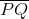. Without measuring, construct a copy of.

Solutions:

(1) Let the given line segment be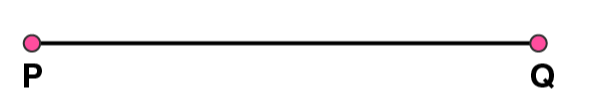(2) Adjust the compasses up to the length of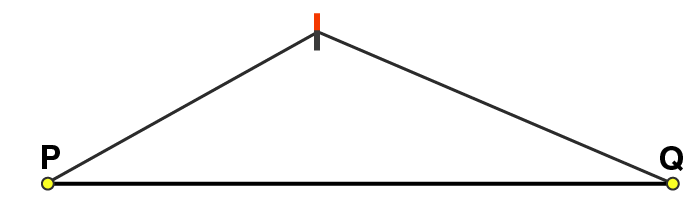(3) Draw any line l and mark a point A on it(4) Place the pointer on point A, without changing the setting of compasses, draw an arc to cut the line segment at B point.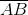is the required line segment

2. Given some line segment, whose length you do not know, constructsuch that the length ofis twice that of.

Solutions:

Following steps are followed to construct a line segmentsuch that the length ofis twice that of(1) Let the given line segment be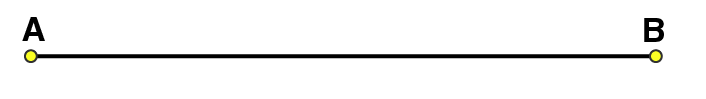(2) Adjust the compasses up to the length of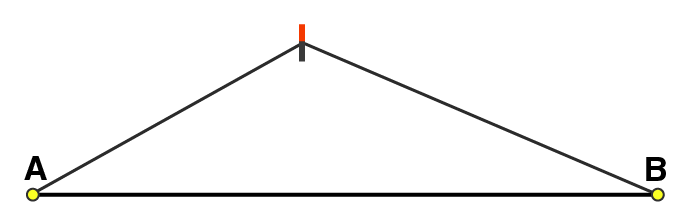(3) Draw a line l and mark a point P on it(4) Place the pointer on P, draw an arc to cut the line segment at point X, without changing the setting of compasses(5) Again with same radius as before, by placing the pointer on point X, draw an arc to cut the line l at point Qis the required line segment.